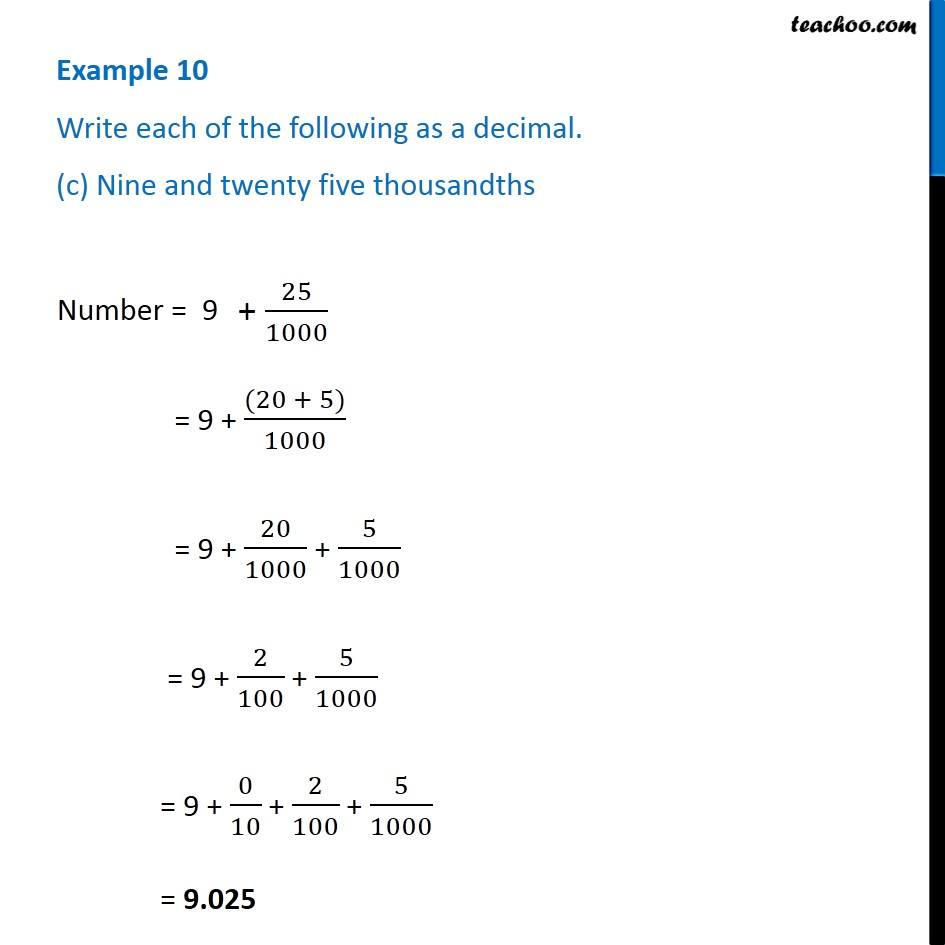Examples

Chapter 8 Class 6 Decimals
Serial order wiseLearn in your speed, with individual attention - Teachoo Maths 1-on-1 Class

### Transcript

Example 10 Write each of the following as a decim(c) Nine and twenty five thousandthsNumber = = 9 + ((20 + 5))/1000 = 9 + 20/1000 + 5/1000 = 9 + 2/100 + 5/1000 = 9 + 0/10 + 2/100 + 5/1000 = 9.025